# Intuition behind the Hungarian Algorithm

The Hungarian Algorithm (see here, here, or here) is an easy-to-perform method for solving an assignment problem, a well-studied problem in the field of combinatorial optimization. An example of a simple assignment problem is the following: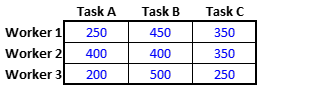Example: A company wants to temporarily hire 3 workers to do 3 tasks at the same time. The cost each worker charges to do each task is as shown in the table. How should the company assign the workers to the tasks to minimize its total cost?

Here is a summary of the Hungarian Algorithm when $m$ assignments are sought:

Step 0. Initialize by forming the $m \times m$ reduced cost matrix from the $m \times m$ cost matrix as follows: For each row, subtract the minimum matrix element in the row from all elements in the row. For each column, subtract the minimum matrix element in the column from all elements in the column.

Step 1. Draw the minimal number of horizontal and vertical lines to cover all zeros in the matrix. If the number of lines $= m$, STOP: an optimal (least cost) assignment is available from among the zero matrix elements of the final reduced cost matrix. Otherwise, continue to Step 2.

Step 2. Find the minimum uncovered element. Subtract this element from each uncovered element. Add this element to each twice-covered element. (Equivalently, subtract the minimum from all uncovered rows and then add it to all covered columns.) Go to Step 1.

The algorithm is illustrated in the figures below (left to right) for the posed assignment problem (sorry only small figs allowed - click to zoom).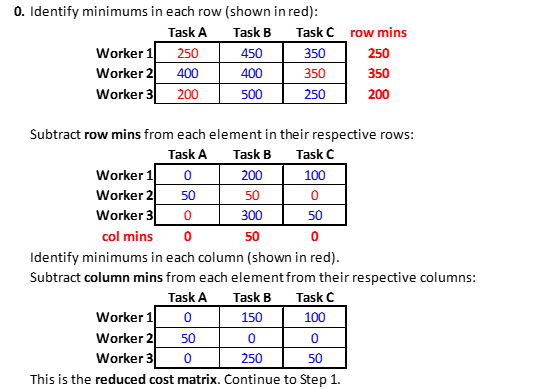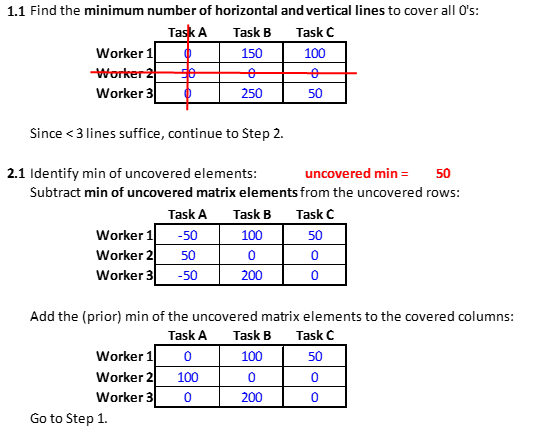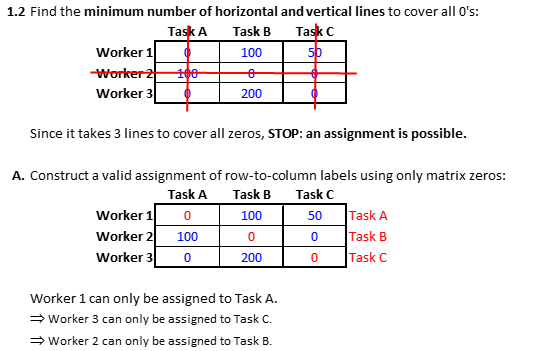The Hungarian Algorithm applied to the example problem $(m=3)$ results in an optimal assignment with a total cost of 900, as can be checked by using brute force to check the total costs of all $m!$ possible assignments. One question that could be asked is why the resulting assignment is optimal, i.e., why does the Hungarian Algorithm work? The purpose of this note is to informally address this question and try to provide intuitive motivation of the algorithm's correctness.

First, write an $m \times m$ assignment problem in the form of a combinatorial optimization problem. Let

\begin{aligned} c_{ij} &\equiv \text{cost of assigning worker } i \text{ to task } j \text{ (the original cost matrix)} \\ x_{ij} &\equiv 1, \text{ if worker } i \text{ is assigned to task } j \text{, and } 0 \text{ otherwise.} \\ \end{aligned}

Then a mathematical programming formulation of the assignment problem is:

\begin{aligned} \min \; &z \\ (1) \qquad &z \equiv \sum_{i=1}^m \sum_{j=1}^m c_{ij} x_{ij} \qquad \qquad \text{ (total cost of an assignment of workers to tasks)}\\ \text{subject to } & \\ (2) \qquad &\sum_{j=1}^m x_{ij} = 1 , \qquad \forall i \in \{1, ..., m\} \qquad \text{ (one task per worker)} \\ (3) \qquad &\sum_{i=1}^m x_{ij} = 1 , \qquad \forall j \in \{1, ..., m\} \qquad \text{ (one worker per task)}\\ (4) \qquad &x_{ij} \in \{0,1\} , \qquad \forall i,j \in \{1, ..., m\} \qquad \text{ (whole assignments only) .}\\ \end{aligned} \;.

Here, the objective function $z$ in (1) is the total cost of assigning all workers to tasks and is to be minimized. The two equality conditions impose the distinct pairing between workers and tasks that makes the workers-to-tasks assignment valid, i.e., exactly one task to each worker (2) and exactly one worker to each task (3). The last condition (4) simply requires assignments to be whole and complete, not partial as in one worker does half of a task and another does the other half (i.e., it is an integer linear program).

Now, as seen in the example, the Hungarian algorithm appears to operate entirely on the cost matrix for the problem. So, suppose we introduce quantities $\{u_i\}$ and $\{v_j\}$ to somehow modify the cost matrix $\{c_{ij}\}$ by adding and subtracting them in $z$ so that the total value of $z$ is unchanged: \begin{aligned} \qquad z &= \sum_{i=1}^m \sum_{j=1}^m c_{ij} x_{ij} \\ &= \sum_{i=1}^m \sum_{j=1}^m ( c_{ij} - u_i - v_j + u_i + v_j) x_{ij} \\ &= \sum_{i=1}^m \sum_{j=1}^m ( c_{ij} - u_i - v_j ) x_{ij} + \sum_{i=1}^m u_i \sum_{j=1}^m x_{ij}+ \sum_{j=1}^m v_j \sum_{i=1}^m x_{ij} \\ &= \sum_{i=1}^m \sum_{j=1}^m ( c_{ij} - u_i - v_j ) x_{ij} + \sum_{i=1}^m u_i \cdot 1 + \sum_{j=1}^m v_j \cdot 1 \qquad \text{ (used conditions (2) and (3) ) } \\ &= \sum_{i=1}^m \sum_{j=1}^m ( c_{ij} - u_i - v_j ) x_{ij} + \sum_{i=1}^m u_i + \sum_{j=1}^m v_j \;. \\ \end{aligned}

From this, the total cost $z$ can be written as $(5) \qquad \qquad z = \sum_{i=1}^m \sum_{j=1}^m c_{ij}^0 x_{ij} + z^0$ where $c_{ij}^0 \equiv c_{ij} - u_i - v_j$ are the modified costs and $z^0 \equiv \sum_{i=1}^m u_i + \sum_{j=1}^m v_j$ is a fixed cost added to the total cost expression. Now, if somehow the values of these quantities $\{u_i\}$ and $\{v_j\}$ are chosen so that $c_{ij} - u_i - v_j = c_{ij}^0 = 0$ for certain row-column indices $(i,j)$ that correspond to a valid assignment, then choosing $x_{ij} = 1$ for these $(i,j)$-indices minimizes z (and therefore solves the assignment problem!). This is because all the resulting $c_{ij}^0 x_{ij}$ terms for $z$ in $(5)$ are 0 since either $c_{ij}^0 =0$ when $x_{ij} = 1$ or $x_{ij} = 0$, in which case $(5)$ reduces to $z = z^0$, a quantity independent of variables $x$, and the minimum value of a constant is just the constant.

What are these mysterious quantities $\{u_i\}$ and $\{v_j\}$?
First observe that they are the sole contributor to the optimal total cost since $z = z^0 = \sum_{i=1}^m u_i + \sum_{j=1}^m v_j$. Second, in the expression $c_{ij} - u_i$ for a fixed $i$, $u_i$ is subtracted from $c_{ij}$ for every $j$; in other words, $u_i$ is the quantity subtracted from row $i$ of the cost matrix $\{c_{ij} \}$. Similarly, $v_j$ is the quantity subtracted from column $j$ of the matrix that results from subtracting $u_i$ from row $i$ of the matrix $\{c_{ij}\}$. The resulting matrix has elements $c_{ij} - u_i -v_j$ (the final reduced cost matrix elements). Therefore, the quantities $\{u_i\}$ and $\{v_j\}$ are the total amounts determined by the Hungarian Algorithm that when subtracted from the rows and columns, respectively, of the original cost matrix will result in an optimal assignment (based on the final reduced cost matrix). It can be verified in the example problem that when all the row, column, and uncovered element minimums are totaled, the result is the minimum cost: 250 + 350 + 200 + 0 + 50 + 0 + 50 = 900! So, the Hungarian Algorithm operation can be thought of as systematically transferring cost to $z^0$ from the original cost matrix for those matrix elements that will end up being candidate costs in a minimum cost assignment.

Hopefully the foregoing suggests why the Hungarian Algorithm gives rise to an optimal assignment. How it comes up with these "subtractors" $\{u_i\}$ and $\{v_j\}$ requires a deeper discussion involving duality theory: every linear program has a companion linear program called its dual that is solved simultaneously, and in the case of the assignment problem, the $\{u_i\}$ and $\{v_j\}$ are the dual variables. Interested readers can consult K. Murty's excellent free online book Network Programming, specifically the first 8 or so pages of Chapter 3. [Note that Prof. Murty's presentation of the Hungarian Algorithm is an equivalent but different version than the above, but it is the discussion leading up to the algorithm presentation you might find particularly insightful].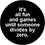Note by Wesley Zumino
3 years, 11 months ago

This discussion board is a place to discuss our Daily Challenges and the math and science related to those challenges. Explanations are more than just a solution — they should explain the steps and thinking strategies that you used to obtain the solution. Comments should further the discussion of math and science.

When posting on Brilliant:

• Use the emojis to react to an explanation, whether you're congratulating a job well done , or just really confused .
• Ask specific questions about the challenge or the steps in somebody's explanation. Well-posed questions can add a lot to the discussion, but posting "I don't understand!" doesn't help anyone.
• Try to contribute something new to the discussion, whether it is an extension, generalization or other idea related to the challenge.

MarkdownAppears as
*italics* or _italics_ italics
**bold** or __bold__ bold
- bulleted- list
• bulleted
• list
1. numbered2. list
1. numbered
2. list
Note: you must add a full line of space before and after lists for them to show up correctly
paragraph 1paragraph 2

paragraph 1

paragraph 2

[example link](https://brilliant.org)example link
> This is a quote
This is a quote
    # I indented these lines
# 4 spaces, and now they show
# up as a code block.

print "hello world"
# I indented these lines
# 4 spaces, and now they show
# up as a code block.

print "hello world"
MathAppears as
Remember to wrap math in $$ ... $$ or $ ... $ to ensure proper formatting.
2 \times 3 $2 \times 3$
2^{34} $2^{34}$
a_{i-1} $a_{i-1}$
\frac{2}{3} $\frac{2}{3}$
\sqrt{2} $\sqrt{2}$
\sum_{i=1}^3 $\sum_{i=1}^3$
\sin \theta $\sin \theta$
\boxed{123} $\boxed{123}$

Sort by:

Hi Wesley -- this is fantastic! Would you mind if we merge some of this content into here? It will leave this discussion unchanged.

Staff - 3 years, 10 months ago

Eli - no prob at all. Actually, I had considered that myself but, being new here, I was concerned about making any changes to Karleigh and Nathan's work that might have to be made to smooth this material in. FYI, the content of this note is original (at least I haven't seen it before), so there are no copyright issues. I did get some inspiration from Murty's text.

And thank you very much for the kind sentiments!

- 3 years, 10 months ago